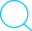16 Mar 2017

# Model-free control algorithms for deep reinforcement learning --Similarities and differences (WIP)

Conventions:

• Sets are represented by $\mathcal{A, S}$,… (caligraphy font)
• Vectors are represented by $\mathbf{w, \theta,…}$ (bold font)
• Functions are represented by $Q,\hat Q, V, \hat V,$… (capital letters)
• Random variables are represened by $s,a,r$… (lower case letters)

Note: This post is for comparing the differences and understanding the similarities of various model-free control algorithms in (deep) reinforcement learning (especially with function approximations). It is not intended to be a primer or a comprehensive refresher. Please refer to Sutton & Barto, 2017 for completeness.

## n-step return for action-value function with value function approximation

$q_{t,\mathbf{w_t}}^{(n)}=r_{t+1}+ \gamma * r_{t+2} + \gamma ^2 * r_{t+3} + ...\gamma^{n-1}*r_{t+n}+\gamma^n * \hat Q(s_{t+n},a_t,\mathbf w_t)$

equivalently,

$q_{t,\mathbf w_t}^{(n)}= \sum_{i=1}^n \gamma^{i-1} * r_{t+i} + \gamma^{i} * \hat Q(s_{t+n},a_t, \mathbf w_t)$

## $\mathbf{\lambda -return}$ for action-value function with value function approximation

$q_{t,\mathbf{w_t}}^{(\lambda)}= (1-\lambda) \sum_{n=1}^{\infty}\lambda^{n-1}q_{t,\mathbf{w_t}}^{(n)}$

## SARSA update with function approximation for on-policy model-free control

At time step $t+1$, the error at timestep $t$ is used to adjust the estimate of $Q(s_t,a_t,\mathbf{w_t})$ through the following weight (of the Q-value function approximator) update:

$\Delta \mathbf{w_{t+1}}= \alpha * [ r_{t+1} + \gamma * \hat Q(s_{t+1}, a_t , \mathbf{w_t}) - \hat Q(s_t,a_t,\mathbf{w_t})] \nabla_w \hat Q(s_t,a_t,\mathbf{w_t})$

This is similar to the $TD(0)$ update step for state-value function.

## One-step Q-learning update with function approximation [[Lin;1992]][]

At time step $t+1$, the error at timestep $t$ is used to adjust the estimate of $Q(s_t,a_t,w_t)$ through the following weight (of the Q-value function approximator) update:

$$\Delta \mathbf{w_{t+1}}= \alpha * [ r_{t+1} + \gamma * \max\limits_{a\in \mathcal A} \hat Q(s_{t+1}, a , \mathbf{w_t}) - \hat Q(s_t,a_t,\mathbf{w_t})] \nabla_w \hat Q(s_t,a_t,\mathbf{w_t})$$ This is similar to the $TD(0)$/ SARSA update step except for the $\max_{a\in\mathcal A}$ on the $\hat Q$ function (which means that the $\hat Q$ value associated with the action that yields the maximum value in state $s_{t+1}$ is used or equivalently, $\hat Q(s_{t+1},argmax_{a’}(\hat Q(s_{t+1},a’)),w)$ ).

## Temporal Difference Q-Learning with function approximation [[Watkins;1989]][]

$\Delta w_t = \alpha [r_{t+1} + \gamma \max_{a \in \mathcal{A}} Q(s_{t+1}, a, w) - Q(s_t, a_t, w)] \sum_{k=0}^t( \lambda \gamma)^{t-k}\nabla_wQ(s_k,a_k,w)$